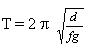Oscillation by Friction
 What is Oscillation by Friction? Simulation by Interactive Physics Formula of the Oscillation Bibliography Comments .
 To the Main Page    "Mathematische Basteleien"

What is Oscillation by Friction?
 ...... If you put a rod on two wheels, which are driven by two equal motors with opposite turns, it starts oscillating.
Explanation
If you move the rod to the left, you put more weight on the left wheel than on the right one. Therefore the force of friction on the left is bigger than on the right. The rod is moved to the right. If the main emphasis is on the middle, the forces neutralize themselves. The rod is moving beyond the centre line because of inertia, until the force of friction is bigger on the right. The rod is moving to the left. - The rod is oscillating.

This arrangement can be set up quickly. You can use any motor, also those from  LEGO. You can use a pencil as a rod.

Simulations with 'Interactive Physics'   top
You can simulate this experiment with the computer program "Interactive Physics". Use a rectangle and two motors with two circles on them. (I used the standard settings as much as possible). The following screen is produced.Formula of the Oscillation top There are sine oscillations. A calculation confirms this: The linear law of forces is valid. Hence you have an harmonic motion. The formula for the period is:T is the period, 2d the distance of the axes of the motors, f the value of kinetic friction and g the acceleration due to gravity. It is remarkable that the period is independant of the mass of the rod.  In practice you have a light increase of the period with the mass: The motors turn less, if you have weight. If you always regulate the motor to the same frequency with the help of stroboscope lightning, the supporting surface becomes warm. f is changing. - You can prove the dependance T=T(d) to your satisfaction. Experiments with friction are delicate. You can measure value of kinetic friction directly, if the motors run in the same direction and if you hold on the rod by a dynamometer.

Bibliography    top
Gamow, Cleveland: Physik in unserer Welt 1, München 1963
Karl-Heinz Gronemeier, Otto Kranz, Hans Steidel: Fachmethodische Arbeiten zur Mechanik, Shaker Verlag, Aachen 1999

The experiment is worthy of being more well-known. The experiment is simple. The mathematics to this experiment are elementary.
This is another example of an harmonic motion, which belongs to the most famous phenomenons of physics.

Feedback: Email address on my main page